# Create Heatmap in R Using ggplot2

• Last Updated : 29 Jul, 2021

A heatmap depicts the relationship between two attributes of a dataframe as a color-coded tile. A heatmap produces a grid with multiple attributes of the dataframe, representing the relationship between the two attributes taken at a time.

Dataset used: bestsellers

Let us first create a correlation matrix to understand the relation between different attributes, for this cor() function is used.

Syntax: cor(dataframe)

Note: This function fails when the dataframe consists of values apart from numeric values, so we will also use the sapply() method.

Example:

## R

 `df <- ``read.csv``(``"bestsellers.csv"``)` `cor``(df[``sapply``(df, is.numeric)])`

Output:Now that we have a correlation matrix, we have to melt it in a form that a heatmap can be created. For this melt() function of reshape2 library is used.

Melting in R programming is done to organize the data. It is performed using melt() function which takes dataset and column values that have to be kept constant. Using melt(), dataframe is converted into a long format and stretches the data frame.

Syntax: melt(data, na.rm = FALSE, value.name = “value”)

Parameters:

• data: represents dataset that has to be reshaped
• na.rm: if TRUE, removes NA values from dataset
• value.name: represents name of variable used to store values

Example:

## R

 `library``(ggplot2)``library``(reshape2)` `df <- ``read.csv``(``"bestsellers.csv"``)` `data <- ``cor``(df[``sapply``(df,is.numeric)])` `data1 <- ``melt``(data)` `head``(data1)`

Output: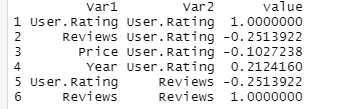To create a heatmap with the melted data so produced, we use geom_tile() function of the ggplot2 library. It is essentially used to create heatmaps.

Syntax: geom_tile(x,y,fill)

Parameter:

• x: position on x-axis
• y: position on y-axis
• fill: numeric values that will be translated to colors

To this function, Var1 and Var2 of the melted dataframe are passed to x and y respectively. These represent the relation between attributes taken two at a time. To fill parameters provide, since that will be used to color-code the tiles based on some numeric value.

Example:

## R

 `library``(ggplot2)``library``(reshape2)` `df <- ``read.csv``(``"bestsellers.csv"``)` `data <- ``cor``(df[``sapply``(df,is.numeric)])` `data1 <- ``melt``(data)` `ggplot``(data1, ``aes``(x = Var1,``                  ``y = Var2,``                  ``fill = value))+``geom_tile``()`

Output: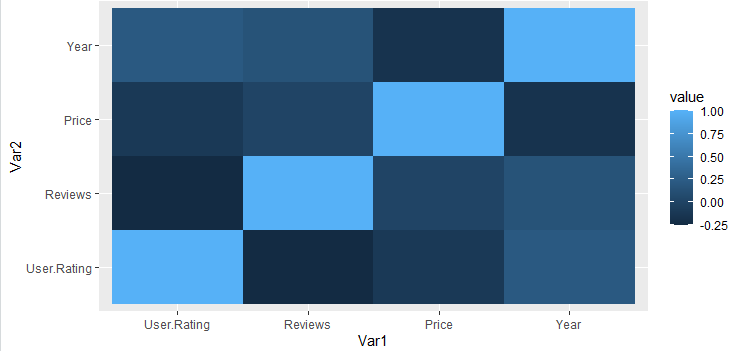### Changing color:

#### The color of the plot can be changed using three functions:

Syntax:

Parameter:

• low: color to highlight smaller values
• high: color to highlight bigger values

## R

 `library``(ggplot2)``library``(reshape2)` `df<-``read.csv``(``"bestsellers.csv"``)` `data<-``cor``(df[``sapply``(df,is.numeric)])` `data1<-``melt``(data)` `ggplot``(data1,``aes``(x = Var1, y = Var2, fill = value))+``geom_tile``()+``scale_fill_gradient``(high = ``"green"``, low = ``"white"``)`

Output: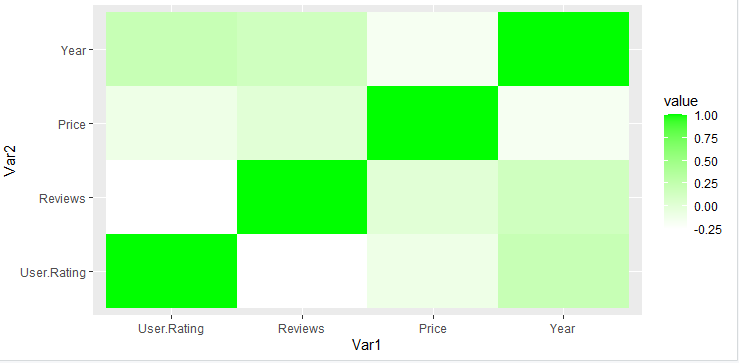• scale_fill_distiller(): It used to customize according to ColorBrewer palette.

Syntax: scale_fill_distiller(palette)

## R

 `library``(ggplot2)``library``(reshape2)` `df<-``read.csv``(``"bestsellers.csv"``)` `data<-``cor``(df[``sapply``(df, is.numeric)])` `data1<-``melt``(data)` `ggplot``(data1,``aes``(x = Var1, y = Var2,fill = value))+``geom_tile``() + ``scale_fill_distiller``(palette = ``"Spectral"``)`

Output: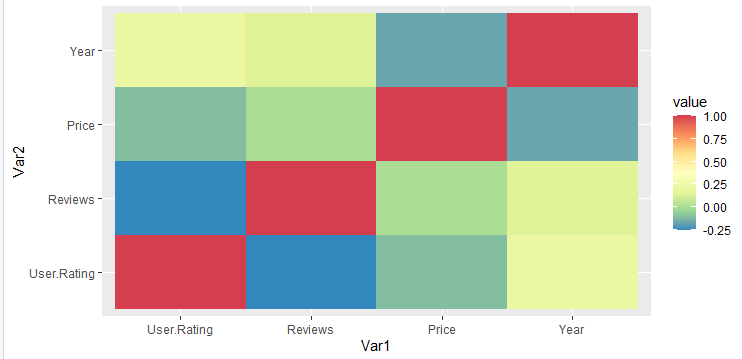• scale_fill_viridis(): to use viridis. In this function, discrete is set to FALSE.

Syntax: scale_fill_viridis(discrete)

## R

 `library``(ggplot2)``library``(reshape2)``library``(viridis)` `df<-``read.csv``(``"bestsellers.csv"``)` `data<-``cor``(df[``sapply``(df,is.numeric)])` `data1<-``melt``(data)` `ggplot``(data1, ``aes``(x = Var1, y = Var2, fill = value))+``geom_tile``() + ``scale_fill_viridis``(discrete = ``FALSE``)`

Output: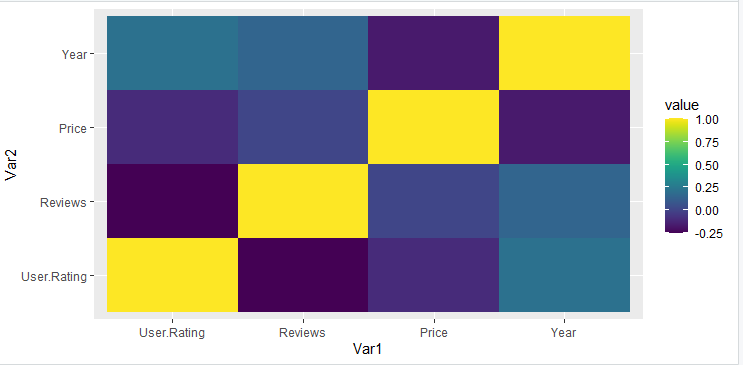### Order the row:

A heatmap can be reordered by reordering its y-elements. This can be done by reorder().

Syntax: reorder(y_value,value)

Where, Value is the element to reorder by.

## R

 `library``(ggplot2)``library``(reshape2)` `df<-``read.csv``(``"bestsellers.csv"``)` `data<-``cor``(df[``sapply``(df,is.numeric)])` `data1<-``melt``(data)` `ggplot``(data1,``aes``(x = Var1, y = ``reorder``(Var2, value),``                 ``fill = value)) + ``geom_tile``()`

Output: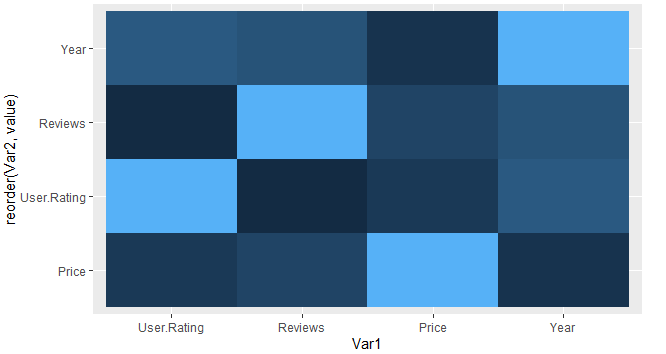The title can be added to a heatmap to make it descriptive. It can be done by using ggtitle().

Syntax: ggtitle(“title”)

## R

 `library``(ggplot2)``library``(reshape2)` `df<-``read.csv``(``"bestsellers.csv"``)` `data<-``cor``(df[``sapply``(df,is.numeric)])` `data1<-``melt``(data)` `ggplot``(data1, ``aes``(x = Var1, y = Var2, fill = value))+``geom_tile``()+``ggtitle``(``"Bestsellers"``)`

Output: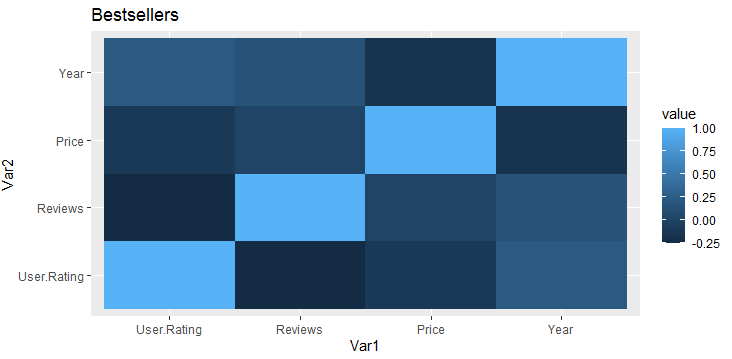### Removing Labels:

Labels of the heatmap can also be removed to show only the corresponding values it is representing. If we remove labels, keeping ticks doesn’t make sense. We can use attributes of theme() function axis.ticks and axis.text and set them to element_blank().

Syntax: theme(axis.ticks = element_blank(), axis.text = element_blank())

## R

 `library``(ggplot2)``library``(reshape2)` `df<-``read.csv``(``"bestsellers.csv"``)` `data<-``cor``(df[``sapply``(df,is.numeric)])` `data1<-``melt``(data)` `ggplot``(data1,``aes``(x=Var1,y=Var2,fill=value))+``geom_tile``()+``  ``theme``(axis.ticks = ``element_blank``(),``        ``axis.text = ``element_blank``())`

Output: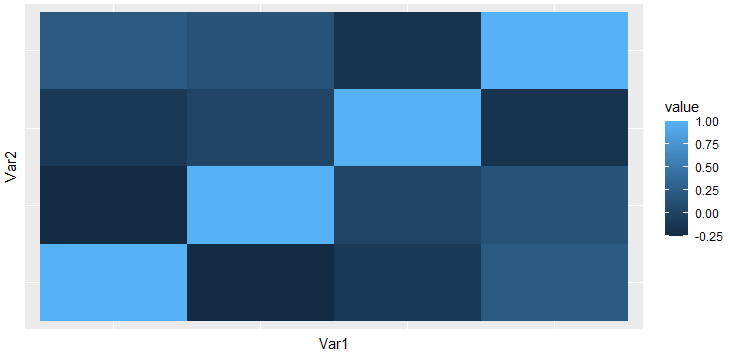My Personal Notes arrow_drop_up